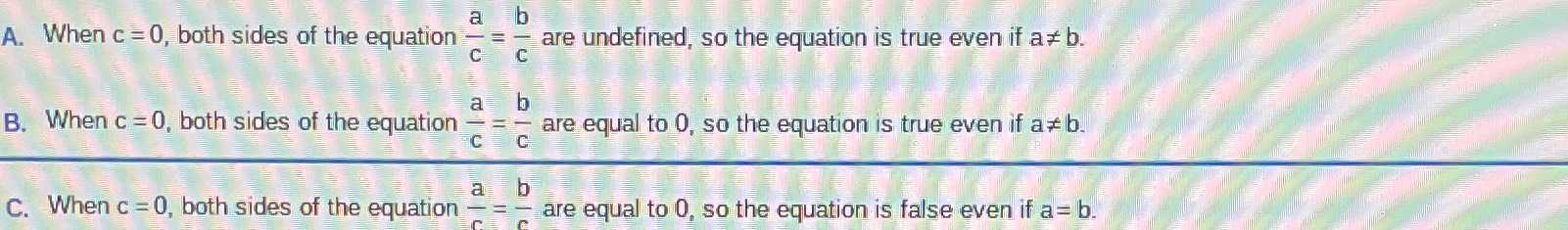### ¿Todavía tienes preguntas de matemáticas?

Pregunte a nuestros tutores expertos
Algebra
PreguntaA. When $$c = 0$$ , both sides of the equation $$\frac { a } { c } = \frac { b } { c }$$ are undefined, so the equation is true even if $$a \neq b$$ .

B. When $$c = 0$$ , both sides of the equation $$\frac { a } { c } = \frac { b } { c }$$ are equal to $$0$$ , so the equation is true even if $$a \neq b$$ .

C. When $$c = 0$$ , both sides of the equation $$\frac { a } { c } = \frac { b } { c }$$ are equal to $$0 ,$$ so the equation is false even if $$a = b$$ .